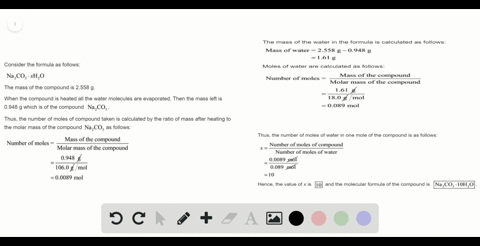Sign up for our free STEM online summer camps starting June 1st!View Summer Courses### Valproic acid, used to treat seizures and bipolar…

01:27Auburn University Main Campus

Need more help? Fill out this quick form to get professional live tutoring.

Get live tutoring
Problem 56

(a) The characteristic odor of pineapple is due to ethyl butyrate, a compound containing carbon, hydrogen, and oxygen. Combustion of 2.78 mg of ethyl butyrate produces 6.32 $\mathrm{mg}$ of $\mathrm{CO}_{2}$ and 2.58 $\mathrm{mg}$ of $\mathrm{H}_{2} \mathrm{O} .$ What is the empirical formula of the compound? (b) Nicotine, a component of tobacco, is composed of $C, H,$ and $N .$ A 5.250 -mg sample of nicotine was combusted, producing 14.242 $\mathrm{mg}$ of $\mathrm{CO}_{2}$ and 4.083 $\mathrm{mg}$ of $\mathrm{H}_{2} \mathrm{O} .$ What is the empirical formula for nicotine? If nicotine has a molar mass of $160 \pm 5 \mathrm{g} / \mathrm{mol},$ what is its molecular formula?

a) $\mathrm{C}_{3} H_{6} O$
b) Above

## Discussion

You must be signed in to discuss.